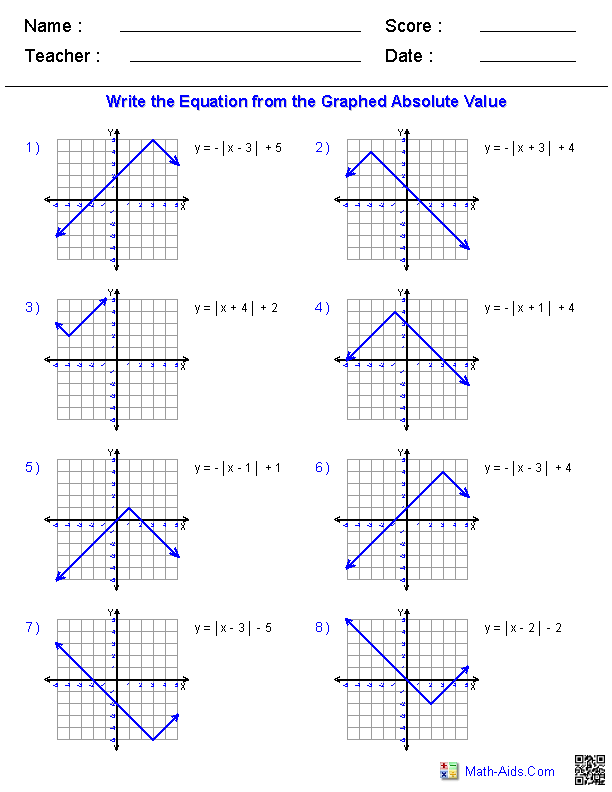#Algebra 2 Worksheets

## Linear Functions Worksheets

Here is a graphic preview for all of the Linear Functions Worksheets. You can select different variables to customize these Linear Functions Worksheets for your needs. The Linear Functions Worksheets are randomly created and will never repeat so you have an endless supply of quality Linear Functions Worksheets to use in the classroom or at home.

Our Linear Functions Worksheets are free to download, easy to use, and very flexible.

These Linear Functions Worksheets are a good resource for students in the 8th Grade through the 12th Grade.

Click here for a Detailed Description of all the Linear Functions Worksheets.

## Quick Link for All Linear Functions Worksheets

Click the image to be taken to that Linear Functions Worksheets.

##### Working withLinear Equations Worksheets##### Graphing Linear InequalitiesLinear Equations Worksheets##### Graphing Absolute ValuesFunctions from EquationsRecommended Videos

## Detailed Description for All Linear Functions Worksheets

Working with Linear Equations Worksheets
These Algebra 2 Linear Equations Worksheets will produce problems for practicing solving the equation of a linear equation. These Linear Equations Worksheets are a good resource for students in the 8th Grade through the 12th Grade.

Graphing Linear Inequalities Worksheets
These Algebra 2 Linear Equations Worksheets will produce problems for practicing graphing linear inequalities. These Linear Equations Worksheets are a good resource for students in the 8th Grade through the 12th Grade.

Graphing Absolute Values Worksheets
These Algebra 2 Linear Equations Worksheets will produce problems for practicing graphing absolute values. These Linear Equations Worksheets are a good resource for students in the 8th Grade through the 12th Grade.